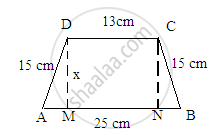Share

# Find the area of a trapezium whose parallel sides are 25 cm, 13 cm and the other sides are 15 cm each. - Mathematics

Course
ConceptArea of Trapezium

#### Question

Find the area of a trapezium whose parallel sides are 25 cm, 13 cm and the other sides are 15 cm each.

#### Solution

Given:
Parallel sides of a trapezium are 25 cm and 13 cm.
Its nonparallel sides are equal in length and each is equal to 15 cm.
A rough skech of the trapezium is given below:\In above figure, we observe that both the right angle triangles AMD and BNC are similar triangles.
This is because both have two common sides as 15 cm and the altitude as x and a right angle.
Hence, the remaining side of both the triangles will be equal.
∴ AM=BN
Also MN=13
Now, since AB=AM+MN+NB:
∴ 25=AM+13+BN
AM+BN=25-13=12 cm
Or, BN+BN=12 cm (Because AM=BN)
2 BN=12
$BN=\frac{12}{2} = 6cm$
∴ AM=BN=6 cm
Now, to find the value of x, we will use the Pythagorian theorem in the right angle triangle AMD whose sides are 15, 6 and x.
${(\text{ Hypotenus })}^2 = (\text{ Base })^2 + (\text{ Altitude })^2$
$(15 )^2 = (6 )^2 + (x)2$
$225 = 36 + x^2$
$x^2 = 225 - 36 = 189$
$\therefore x =\sqrt{189}=\sqrt{9 \times 21}= 3\sqrt{21}cm$
$\therefore\text{ Distance between the parallel sides }=3\sqrt{21} cm$
$\therefore\text{ Area of trapezium }=\frac{1}{2} \times(\text{ Sum of parallel sides })\times(\text{ Distance between the parallel sides })$
$= \frac{1}{2} \times(25+13)\times( 3\sqrt{21})$
$= 57\sqrt{21} {cm}^2$

Is there an error in this question or solution?

#### APPEARS IN

RD Sharma Solution for Mathematics for Class 8 by R D Sharma (2019-2020 Session) (2017 to Current)
Chapter 20: Mensuration - I (Area of a Trapezium and a Polygon)
Ex. 20.2 | Q: 17 | Page no. 24

#### Video TutorialsVIEW ALL 

Solution Find the area of a trapezium whose parallel sides are 25 cm, 13 cm and the other sides are 15 cm each. Concept: Area of Trapezium.
S